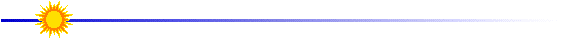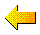# Scaling the Yohkoh BlueprintsIf you would like to scale the Yohkoh blueprint to exactly the size of your box this is how to do it. Go to the box measurement page and choose the option for a 45 cm box. (This is a good length because each blueprint fits on one page.)

Go through the lesson as if you were making a satellite that is 45 cm long. BUT, each time you print out an instrument blueprint you will need to scale that blueprint by the following amount.

Take the length of your box in cm, divide that length by 45 and multiply that number by 100. The result you get is the percentage you need to scale each blueprint by. For instance, if you have a 60 cm box you would need to scale each blueprint by (60/45)*100 = 133%

You can do the scaling on a copy machine. Another method is to multiply each segment of the blueprint by the number you get when you divide your box length by 45. You could then redraw the blueprint by hand.

For example, if you had a 60 cm box you would multiply each segment of the blueprint by 1.33. In this case a 30mm line would be redrawn to ~40 mm.

If you do not want to rescale all the blueprints, chose the box length from the menu on the previous page that is smaller than your box and you will be just fine!Enter your box lengthModel Satellite Lesson